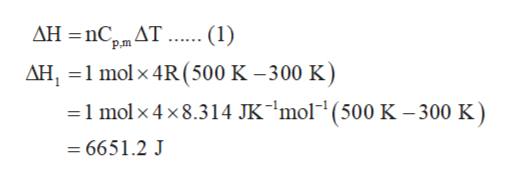# Use the molar constant volume heat capacities for gases given in Topic 4C (as multiples for R) to estimate the change in reaction enthalpy of N2 (g) + 3 H2 (g) ---> 2 NH3 (g) when the temperture is increased from 300. K to 500. K. Ignore the vibrational contributions to heat capacity. Is the reaction more or less exothermic at the higher temperature?  AtomsLinear MoleculesNonlinear MoleculesCv,m 3/2 R5/2 R3 RCp,m5/2 R7/2 R4 R

Question
146 views

Use the molar constant volume heat capacities for gases given in Topic 4C (as multiples for R) to estimate the change in reaction enthalpy of N2 (g) + 3 H2 (g) ---> 2 NH3 (g) when the temperture is increased from 300. K to 500. K. Ignore the vibrational contributions to heat capacity. Is the reaction more or less exothermic at the higher temperature?

 Atoms Linear Molecules Nonlinear Molecules Cv,m 3/2 R 5/2 R 3 R Cp,m 5/2 R 7/2 R 4 R

check_circle

Step 1

The change in enthalpy (ΔH) of each reactant and product is calculated using equation (1). The molar constant pressure heat capacity (Cp,m) for NH3 is 4R. The change in enthalpy of NH3 (ΔH1) is calculated as follows:help_outlineImage TranscriptioncloseΔΗ-nC,m ΔΤ (1) ΔΗ, -1 mol x 4R (500 K -300 K) -1 molx 4 x8.314 K'mol"' (500 Κ - 300 K) = 6651.2 J fullscreen
Step 2

The molar constant pressure heat capacity (Cp,m) for N2 is 7/2R. The change in enthalpy of N2 (ΔH2) is calculated as follows:

Step 3

The molar constant pressure heat capacity (Cp,m) for H2 is 7/2R. The change in enthalpy...

### Want to see the full answer?

See Solution

#### Want to see this answer and more?

Solutions are written by subject experts who are available 24/7. Questions are typically answered within 1 hour.*

See Solution
*Response times may vary by subject and question.
Tagged in

### Chemical Thermodynamics